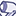# QlikView App Dev

Discussion Board for collaboration related to QlikView App Development.

Announcements
Live chat with experts, bring your API Integration questions. June 15th, 10 AM ET. REGISTER TODAY
cancel
Showing results for
Search instead for
Did you mean:Contributor III

## Add expression into list box

Hi everyone,

I have created an expression in a straight table and i would like to add this into a list box so that users are able to filter via the list box.

I have tried numerous ways to add this expression but i know that it wont accept TOTAL.

Is the only way to achieve this by doing a GROUP BY in my script? Will this not affect all of my query?

Here is my expression:

if(sum(TOTAL <[SWIFT ID]>RV_WEEKLY_COST) >'250.00' and

sum(TOTAL <[SWIFT ID]>RV_WEEKLY_COST) <='500.00','2) £250 - £500',

if(sum(TOTAL <[SWIFT ID]>RV_WEEKLY_COST) >='0.00' and

sum(TOTAL <[SWIFT ID]>RV_WEEKLY_COST) <='250.00','1) < £250'))

Any help is much appreciatedThanks

1 Solution

Accepted SolutionsMVP

Try like:

Aggr(

if(sum(TOTAL <[SWIFT ID]>RV_WEEKLY_COST) >'250.00' and

sum(TOTAL <[SWIFT ID]>RV_WEEKLY_COST) <='500.00','2) £250 - £500',

if(sum(TOTAL <[SWIFT ID]>RV_WEEKLY_COST) >='0.00' and

sum(TOTAL <[SWIFT ID]>RV_WEEKLY_COST) <='250.00','1) < £250'))

, dim1, dim2..)

dim1, dim2.. would be your chart dimensions that the aggregation is carried out against.

3 RepliesMVP

Try like:

Aggr(

if(sum(TOTAL <[SWIFT ID]>RV_WEEKLY_COST) >'250.00' and

sum(TOTAL <[SWIFT ID]>RV_WEEKLY_COST) <='500.00','2) £250 - £500',

if(sum(TOTAL <[SWIFT ID]>RV_WEEKLY_COST) >='0.00' and

sum(TOTAL <[SWIFT ID]>RV_WEEKLY_COST) <='250.00','1) < £250'))

, dim1, dim2..)

dim1, dim2.. would be your chart dimensions that the aggregation is carried out against.MVP

If what tresesco‌ gave doesn't work, would you be able to share a sample?Contributor III
Author

Yes it works!

Thank you so much for your help